# Numb3rs 218: All's Fair

Sudoku and logistic regression. They have two things in common: both appeared in this episode of Numb3rs, and both involve some interesting math. What math? Great question! Read on and find out.

## Squares within Squares

Although Sudoku first became popular in Japan, it was in fact invented by an American, Howard Garns, in 1979. The goal is to place the numbers 1 through 9 in square cells of a 9x9 board such that1. Each row and column includes each digit.
2. Each of the smaller 3x3 squares also includes each digit.
Usually some numbers are filled in to begin with, and you must fill in the rest. Here's one courtesy of wikipedia: give it a try, it's fun! You can find more Sudoku puzzles at http://www.dailysudoku.com/. Although Sudoku is a recent phenomenon, the mathematical object on which it's based, the Latin square, is much older. As the name implies, a Latin square is a square grid, say nxn in size, such that only the first condition above is satisfied, i.e. each row and each column must have a cell with each of n different numbers or symbols.

Activity 1:
1. Construct a 2x2 Latin square. Ok, now construct a different 2x2 Latin square. Can you make another one, different from the first two?
2. Complete the following 4x4 Latin square (with digits 1, 2, 3, and 4):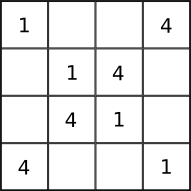Activity 2: Notice that when you switch two rows or columns of a Latin square, you still get a Latin square. We call two Latin squares non-isomorphic if you can't get one from the other by switching (perhaps multiple) rows or columns. How many non-isomorphic 3x3 Latin squares (with entries 1, 2, and 3) are there?

One of the first seriously study the mathematics of Latin squares was the prolific 18th century mathematician Leonhard Euler (OIL-ler), who was intrigued by the following puzzle: given six different regiments that each have six officers, each of one of six different ranks, can these 36 officers be arranged in a 6x6 square so that each row and column has one officer of each rank and regiment? To make this clear, let's mark each regiment by a different color, and denote each of the six ranks by the corresponding die side (see picture below). The question then becomes, can one arrange the dice on a 6x6 board such that each row and column has a die of each color and face value?Activity 3: Can you solve the analogous 2x2 colored die problem? What about 3x3 and 4x4? Here's is a 5x5 solution: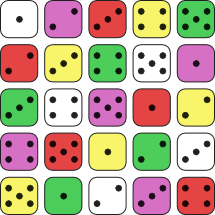In trying to solve the original 6x6 officers puzzle, Euler noticed that the resulting square would break up into two Latin squares, a square of colors and a square of dice in our example.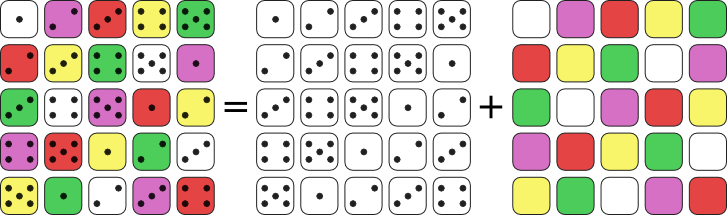Thus all one needs to do to solve the six regiments problem is find a pair of Latin squares which when put together satisfy Euler's requirements, i.e. when the squares are superimposed, each pair of symbols (or die values and colors) occurs exactly once in the whole square. Such pairs are called Graeco-Latin squares, for the simple reason that Euler denoted the elements of one by Latin letters and the other by Greek. After some tinkering, Euler wasn't able to find a 6x6 Graeco-Latin pair, and he conjectured that such pairs don't exist for any n=4k+2, where k is an integer bigger than 0. It wasn't until 1901 that a mathematician showed, by exhaustive enumeration of 6x6 Latin squares, that a 6x6 Graeco-Latin pair truly doesn't exist. Euler's overall conjecture, however, was shown to be false in 1959: Graeco-Latin pairs exist for all n except 2 and 6.

Consider the pair of Latin squares below. Are they Graeco-Latin? We make a single 4x4 square by adding the corresponding entries of the pair. The result is called a magic square. It's a square with distinct integer entries such that the sum of each row, column, and diagonal is the same, in this case 34.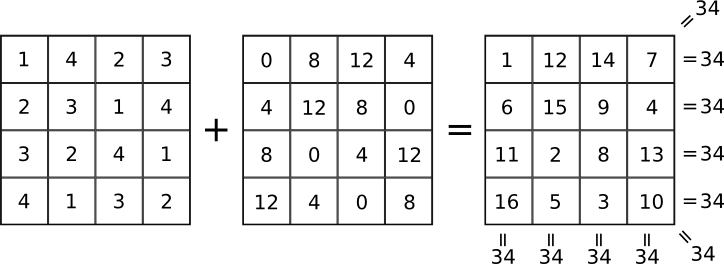Activity 4:
1. What does the sum 1+2+...+n equal, in terms of n?
2. Every magic square of size nxn with entries from 1 to n2 has the same row/column/diagonal sum. For example, in every 4x4 magic square, that sum, called the magic sum, is 34. Construct a 3x3 magic square with entries from 1 to 9 and find its magic sum.
3. What is the magic sum of an nxn magic square (with entries 1 to n2), in terms of n? (Hint: use your answer to part 1.)
Activity 5*: Can you always decompose a magic square into a sum of a Graeco-Latin pair like above? Why or why not? (Hint: recall that no 6x6 Graeco-Latin pairs exist.)
In closing this exploration, here are two basic questions about magic squares which, as far as I know, still have not been answered:
• Can every magic square (with possibly non-consecutive integer entries) be written as a sum of Latin squares? (The answer is no if you restrict yourself to only Graeco-Latin pairs, see activity 5 above.)
• What is the number of different magic squares of size nxn and entries 1 through n2 for n>5?Perhaps the most famous magic square is the one reproduced below. This 4x4 square appears in Albercht Dürer's 1514 engraving Melencolia I, to the right. What other mathematical objects can you find in Dürer's engraving? What do you think is the idea Dürer tried to convey?## One Curve Fits All

As you may already know, The Acme Corporation's yearly production of anvils depends heavily on the amount of iron Acme mines. Here's a data table summarizing the last decade: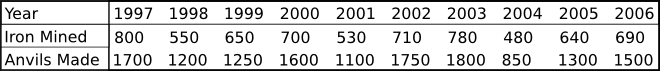Unfortunately, Acme's mining business is in some trouble this year, and is expected to produce only 460 tonnes of iron. Naturally, Acme's CEO wants to know how many anvils can the factory expect to produce, and you've been given the assignment of figuring this out. The first thing you decide to do is graph the points, like this.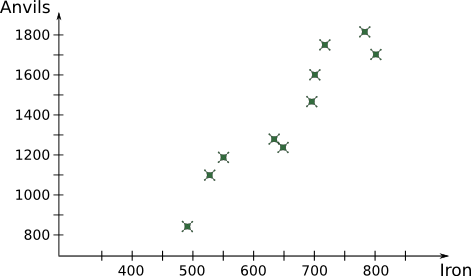After some thought you come up with the following bright idea: all that's needed is a curve, based somehow on the existing data, that will show the correspondence between iron mined and anvils produced.

Activity 1: One possibility is to simply connect the dots in the order of increasing iron, like in the graph to the right.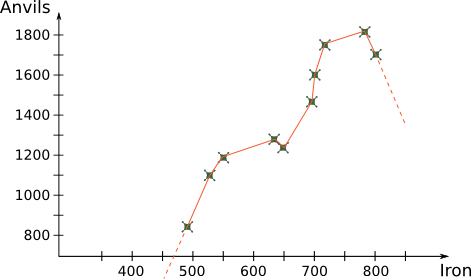1. What are some disadvantages of doing so?
2. Do you think this model will better predict interpolated points, those that fall inside the range of existing data, or extrapolated points, those that fall outside of that range?After trying the above naive approach, you consult a book on statistics and find the method of linear regression. The essence of this method is to fit a line to the existing data points so as to minimize the squares of the vertical distances between the line and the points (see illustration to the right). Why the squares of distances and not simply the distances? Mostly out of mathematical convenience irrelevant to this discussion, details which you'll likely cover in an intermediate level statistics class.

An equation of a line in the plane has the form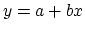for some y-intercept a and slope b. What we would like to do is find a way to write the slope b and intercept a of our regression line in terms of the given data points (xi,yi) (in our case indexed by i=1997, 1998,...,2006). In fact, using a bit of calculus it can be shown that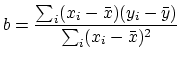and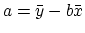where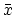is the average of the xi and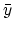is the average of yi. Keep in mind that while you can always fit a regression line to a set of data, the line might not tell you much if the points of your data are not "close" to being on a single line but are scattered all over; it will have no predictive power. On the other hand, in our case of anvils and iron, there's a pretty good linear fit. There's an exact way to quantify how well a regression line fits the data, but that's a story for another day (or wikipedia).

In this episode, Charlie proposes to find how likely someone is to commit a terrorist act based on various variables (money, fanaticism, etc.) using logistic regression. In general, logistic, as opposed to linear, regression is used when you're dealing with a binary--yes/no, pass/fail, 0/1, etc.--dependent variable (the variable you're trying to predict based available data). Here's an example that will make this clear.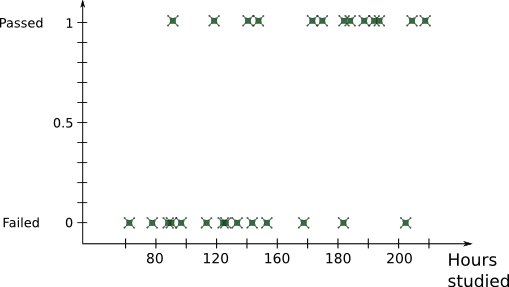Suppose you would like to know how likely a young law school graduate is to pass the bar exam based on how much time they spent studying for it. Further suppose you have the relevant data from last year's 27 exam takers, graphed on the right with pass and fail on the y-axis, and the hours spent studying on the x-axis.

Activity 2: You would like to know what are the chances of somebody who has studied only 110 hours of passing.1. One way to approach this would be to simply do linear regression. It'll look like the picture on the right. What are some problems with this approach?
2. Can you think of a better way of approximating a binary dependent variable by a curve?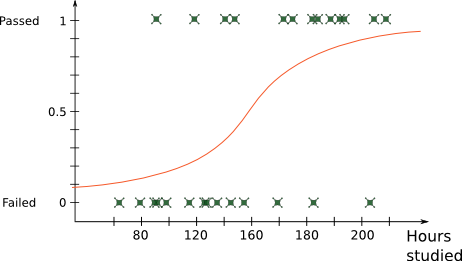In the 1970's statisticians were mulling over question #2 above, and what they came up with is logistic regression. The idea is to approximate the data by an "S" shaped curve, called logistic curve, that looks something like the curve on the right, and is given by the following equation,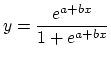where e is the base of the natural logarithm, and a and b are again parameters, but this time not as simple to interpret as intercept and slope in case of linear regression.

Activity 3:
1. How does the logistic curve look like when b=0? What about b>0 or b<0?
2. What are the asymptotes of the curve?

A good way to think about logistic regression, and a very good way to actually approximate it, is as linear regression in disguise, the disguise being the natural log function.

The odds of an event A, say flipping a coin and getting tails, is the probability of it happening divided by the probability of it not happening,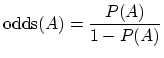Thus if we know the odds of an exam taker passing after studying 120 hours, we can calculate the probability of passing through simple algebra. So without any loss of information, we shift to working with the odds instead of the probability, thinking of odds as a function of events, just like we treated P.

Activity 4:
1. What is the range of values odds can take? (Remember, the range of P is from 0 to 1.)
2. What's the relationship between odds of an event A and the odds of event (not A)?
3. Let log odds(A)=log(P(A)/1-P(A)), where log is the natural logarithm function. What is the range of log odds?
4. What is the relationship between log odds(A) and log odds(not A)?
5. Suppose for some events A and B, P(A) < P(B). Is log odds (A) < log odds(B)?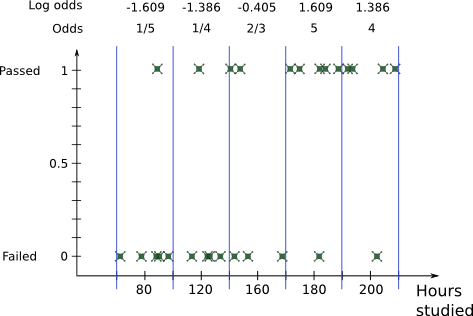As you've seen in part #3 above, the log odds look much more like the continuous dependent variable we used linear regression on earlier in this section. So that's just what we'll do now: use linear regression on the log odds! From our data we can divide the range of hours studied into suitable intervals (of say 40 hours), and count the odds for each interval as (#points at 1)/(#points at 0), as on the right. We then graph the log odds, treating each of the 5 different values we got for the intervals as multiple points according to how many original data points fell into the corresponding interval.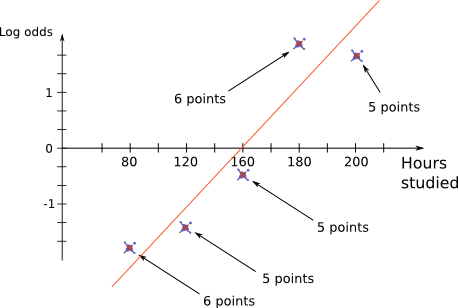Finally, what we get is a regression for the log odds of the form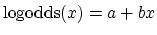as before when we did linear regression. Taking the base of the natural logarithm e to the power of both sides, we get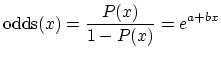and solving for P, we get the equation of the logistic curve!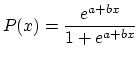Thus the orange regression line to the right is mapped by the transformations above to the logistic curve approximating our original data.

The actual calculation of the logistic curve coefficients is done differently, usually by calculators or computers, but this procedure is simple, conceptually enlightening, and very close to the actual best-fitting logistic curve.

Activity 5:Using the equation for the coefficient b and intercept a of linear regression, find the equation of the regression line approximating the log odds (values of log odds are noted on top of the intervals illustration above). What is the equation of the resulting logistic curve?